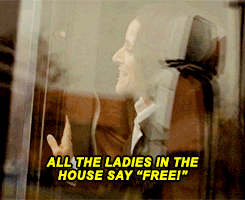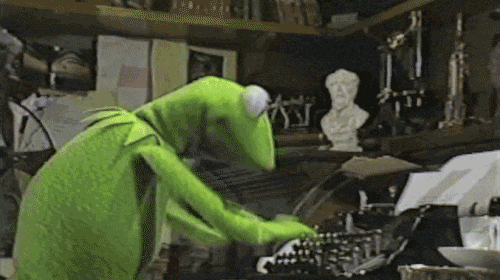# US Wind Farm Locations

Sara Stoudt true
11-12-2018

NOTE: When updating my website in June 2021, this code was revealed to be deprecated. I am using `eval = F` to preserve the post, but code will not run as is. I will try to update at some point (or if you are reading this and now what to do to fix it, let me know).

Tardy as usual…``````library(tidyr)
library(dplyr)
library(ggplot2)
library(geofacet)
library(RColorBrewer)
library(statebins)
setwd("~/Desktop/tidytuesday/data/2018/2018-11-06")
``````

Deal with the missing data: I used `na_if` for the first time here.

``````wind=wind %>% mutate(faa_ors=na_if(faa_ors,"missing"),faa_asn=na_if(faa_asn,"missing"),usgs_pr_id=na_if(usgs_pr_id,-9999), t_state=na_if(t_state,"missing"),t_county=na_if(t_county,"missing"),t_fips=na_if(t_fips,"missing"),p_name=na_if(p_name,"missing"),p_year=na_if(p_year,-9999), p_tnum=na_if(p_tnum,-9999), p_cap=na_if(p_cap,-9999), t_manu=na_if(t_manu,"missing"), t_model=na_if(t_model,"missing"), t_cap=na_if(t_cap,-9999),t_hh=na_if(t_hh,-9999), t_rd=na_if(t_rd,-9999), t_rsa=na_if(t_rsa,-9999),t_ttlh=na_if(t_ttlh,-9999),t_img_date=na_if(t_img_date,"missing"), t_img_srce=na_if(t_img_srce,"missing"))
``````

When windmills became operational by state over time:

``````toP=wind %>% group_by(t_state,p_year) %>% summarise(count=n())
ggplot(toP,aes(p_year,count))+geom_point()+geom_line()+facet_geo(~t_state)
``````

It’s too hard to see what is going on because of big windmill states like CA. Let’s try letting the y-axes be different per state.``````ggplot(toP,aes(p_year,count))+geom_point()+geom_line()+facet_geo(~t_state,scales="free_y")
``````

It’s still hard to see what is going on here. Let’s simplify the question.

total number of windmills per state

Using the version of `statebins` on CRAN…

``````toP2=toP %>% group_by(t_state) %>% summarise(total=sum(count))
toP2=as.data.frame(toP2)
toP2\$t_state=as.character(toP2\$t_state)

library(statebins)
statebins_continuous(toP2,state_col="t_state", value_col="total",brewer_pal="YlOrRd",legend_title="total wind turbines")+guides(fill=guide_colorbar(label.theme=element_text(angle=45,size=10,hjust=1)))
``````

The nice thing about this being built in `ggplot` is that it was easy to add a `guides` statement to rotate the legend labels. I originally took the source code and modified it (in the call to `scale_fill_gradientn`) to create a new function that rotates the legend text. Then I found this that I used below and realized I could just add on to the output of `statebins_continuous` instead of creating a whole new function.

Skewness makes it hard to see what is really going on. How about a transform?

``````toP2\$logTotal=log(toP2\$total)
statebins_continuous(toP2,state_col="t_state", value_col="logTotal",brewer_pal="YlOrRd",legend_title="log(total wind turbines)")+guides(fill=guide_colorbar(label.theme=element_text(angle=45,size=10,hjust=1)))
``````

What year did each state gain the most windmills?

``````toP3=toP %>% group_by(t_state) %>% summarise(maxYear=p_year[which.max(p_year)])
toP3=as.data.frame(toP3)
toP3\$t_state=as.character(toP3\$t_state)
``````

We can’t do the following because we are only allowed up to 9 different categories.

``````statebins(toP3,state_col="t_state", value_col="maxYear",brewer_pal="YlOrRd",legend_title="year w/ most new \n wind turbines",breaks=length(unique(toP3\$maxYear)))
``````

We can use the manual version, but first we have to fix a bug in `statebins_manual` where it assumes `color_col="color"` even though it allows us to pass in something else.

``````colors=c(brewer.pal(9,"YlOrRd"),"black")

key=cbind.data.frame(year=sort(unique(toP3\$maxYear)),colz=colors)

toP3=merge(toP3,key,by.x="maxYear",by.y="year")
toP3\$colz=as.character(toP3\$colz)
``````

Note: we can’t call the variable for color `col` because in the `statebins_manual` function there is a merge to the state/abbreviation key that has a column named `col`. This results in a dataframe with `col.x` and `col.y` as columns, and then when we look to grab color from `col`, we no longer have a column with that name.

``````## get some source files for helper functions
setwd("~/Desktop/statebins/R")
source("aaa.R")
source("util.R")

statebins_manualFix=function(state_data, state_col="state", color_col="color",
text_color="black", font_size=3,
state_border_col="white", labels=NULL,
legend_title="Legend", legend_position="top",
plot_title="", title_position="bottom") {

if (!title_position %in% c("", "top", "bottom")) {
stop("'title_position' must be either blank, 'top' or 'bottom'")
}

state_data <- data.frame(state_data, stringsAsFactors=FALSE)

if (max(nchar(state_data[,state_col])) == 2) {
merge.x <- "abbrev"
} else {
merge.x <- "state"
}

state_data <- validate_states(state_data, state_col, merge.x)

st.dat <- merge(state_coords, state_data, by.x=merge.x, by.y=state_col, all.y=TRUE)
gg <- ggplot(st.dat, aes_string(x="col", y="row", label="abbrev"))
gg <- gg + geom_tile(aes_string(fill=color_col)) ## fixed from fill="color"
gg <- gg + geom_tile(color=state_border_col, aes_string(fill=color_col), size=2, show_guide=FALSE) ## fixed from fill="color"

## allows for a switch to white if block is black and text color is black
if(text_color=="black"){
text_color2=ifelse(st.dat[,color_col]=="black","white","black")
gg <- gg + geom_text(color=text_color2, size=rep(font_size,nrow(st.dat)))

}else{
gg <- gg + geom_text(color=text_color, size=font_size)

}
gg <- gg + scale_y_reverse()
if (is.null(labels)) {
gg <- gg + scale_fill_manual(values=unique(st.dat[,color_col]))
legend_position = "none"
} else {
gg <- gg + scale_fill_manual(values=unique(state_data[,color_col]), labels=labels, name=legend_title)
## switch to unique values from original data set so it matches the order of labels
}
gg <- gg + coord_equal()
gg <- gg + labs(x=NULL, y=NULL, title=NULL)
gg <- gg + theme_bw()
gg <- gg + theme(legend.position=legend_position)
gg <- gg + theme(panel.border=element_blank())
gg <- gg + theme(panel.grid=element_blank())
gg <- gg + theme(panel.background=element_blank())
gg <- gg + theme(axis.ticks=element_blank())
gg <- gg + theme(axis.text=element_blank())

if (plot_title != "") {

if (title_position == "bottom") {
gg <- arrangeGrob(gg, sub=textGrob(plot_title, gp=gpar(cex=1)))
} else {
gg <- gg + ggtitle(plot_title)
}

}

return(gg)

}

statebins_manualFix(toP3,state_col="t_state",color_col="colz",legend_title = "year w/ most new \n wind turbines",legend_position = "top",labels=key\$year,text_color="grey",font_size=5)

## black text unless block is black, then white
statebins_manualFix(toP3,state_col="t_state",color_col="colz",legend_title = "year w/ most new \n wind turbines",legend_position = "top",labels=key\$year,text_color="black",font_size=5)
``````

If we use the updated version that is on GitHub…

First I tried to install both versions and switch between them using this advice, but it didn’t seem to update the `statebins` function.

``````devtools::install_github("hrbrmstr/statebins",lib="/Library/Frameworks/R.framework/Versions/3.5/Resources/library/bonus")
library(statebins, lib.loc="/Library/Frameworks/R.framework/Versions/3.5/Resources/library/bonus")
``````

So I did a hack.``````setwd("~/Desktop/statebins/R")
source("statebins.R")
source("theme-statebin.R")
source("geom-statebins.r")
``````
``````toP3\$maxYear=as.factor(toP3\$maxYear)
#https://stackoverflow.com/questions/6919025/how-to-assign-colors-to-categorical-variables-in-ggplot2-that-have-stable-mappin
names(colors)= levels(toP3\$maxYear)

toP3 %>% statebins(state_col="t_state",
value_col = "maxYear",
dark_label = "white", light_label = "black", ## this is built in now
ggplot2_scale_function = scale_fill_manual,
values=colors,name = "year w/ most new \n wind turbines",font_size=5)+ theme_statebins()

toP2 %>% statebins(state_col="t_state",value_col="total",palette="YlOrRd",name = "log(total wind turbines)")+ theme_statebins() + guides(fill=guide_colorbar(label.theme=element_text(angle=45,size=10,hjust=1)))
``````

My next challenge is to plot points on the map. Because there are wind turbines in Hawaii and Alaska, typical maps in ggplot are incomplete.

If we wanted to create a chloropleth, the `fiftystater` package makes this easy.

``````#devtools::install_github("wmurphyrd/fiftystater")
## couldn't get from CRAN with my R version (3.5.1)

library(fiftystater)

byState <- wind %>% group_by(t_state) %>% summarise(total=n()) %>% mutate(logTotal=log(total))

## Need map_id to be lowercase names
toMatch=cbind.data.frame(abb=state.abb,name=state.name)
byState2=merge(byState,toMatch,by.x="t_state",by.y="abb",all.x=T,all.y=T)
byState2\$name=tolower(byState2\$name)

ggplot(byState2, aes(map_id = name)) +
geom_map(aes(fill = logTotal), map = fifty_states) +
expand_limits(x = fifty_states\$long, y = fifty_states\$lat) +
coord_map() +
scale_x_continuous(breaks = NULL) +
scale_y_continuous(breaks = NULL) +
labs(x = "", y = "") +
theme(legend.position = "bottom",
panel.background = element_blank())+guides(fill=guide_colorbar(label.theme=element_text(angle=45,size=10,hjust=1)))
``````

```

However, if we want to plot points, the scaling for Alaska and Hawaii distorts the rest of the US.

``````library(maps)
all_states <- map_data("state")
p <- ggplot()+ geom_polygon( data=all_states, aes(x=long, y=lat, group = group),colour="black", fill="white" )

p+ geom_point(data=wind,aes(x=xlong,y=ylat))+coord_map(xlim=c(-180,-60), ylim=c(10,70))
``````

To get something that looks like the chloropleth but with points, we need to transform the Alaska and Hawaii data.

``````library(maptools)
tmp_dl <- tempfile()
unzip(tmp_dl, exdir=tempdir())
library(rgdal)

#https://stackoverflow.com/questions/11052544/convert-map-data-to-data-frame-using-fortify-ggplot2-for-spatial-objects-in-r
us_aea <- spTransform(ST, CRS("+proj=laea +lat_0=45 +lon_0=-100 +x_0=0 +y_0=0 +a=6370997 +b=6370997 +units=m +no_defs"))
us_aea@data\$id <- rownames(us_aea@data)

# extract, then rotate, shrink & move alaska (and reset projection)
# need to use state IDs via # https://www.census.gov/geo/reference/ansi_statetables.html
scaleSave = max(apply(bbox(alaska), 1, diff)) / 2.3

# extract, then rotate & shift hawaii
hawaii <- us_aea[us_aea\$STATEFP=="15",]
hawaii <- elide(hawaii, rotate=-35)
hawaii <- elide(hawaii, shift=c(5400000, -1400000))
proj4string(hawaii) <- proj4string(us_aea)

# remove old states and put new ones back in; note the different order
# we're also removing puerto rico in this example but you can move it
# between texas and florida via similar methods to the ones we just used
us_aea <- us_aea[!us_aea\$STATEFP %in% c("02", "15", "72"),]
us50 <- fortify(us_aea, region="STUSPS")

ggplot(data=us50) + geom_map(map=us50, aes(x=long, y=lat, map_id=id, group=group), ,fill="white", color="dark grey", size=0.15)
``````

Now need to transform the data to add to this plot.

``````windT=wind
coordinates(windT) <- ~ xlong + ylat
proj4string(windT) <-CRS(proj4string(ST)) ## this assumes the original

trf=spTransform(windT,CRS(proj4string(us_aea)))

trfRest=subset(trf,!(t_state %in% c("HI","AK") ))
proj4string(trfRest) <- proj4string(trfRest)
hi=subset(trf,t_state =="HI")
ak=subset(trf,t_state=="AK")

ak <- elide(ak, rotate=-50)
ak <- elide(ak, scale=scaleSave)
ak  <- elide(ak, shift=c(-2100000, -2500000))
proj4string(ak) <- proj4string(us_aea)

# extract, then rotate & shift hawaii
hi <- elide(hi, rotate=-35)
hi <- elide(hi, shift=c(5400000, -1400000))
proj4string(hi) <- proj4string(us_aea)

transformed <- rbind(trfRest, ak, hi)

windT <- as.data.frame(transformed)

ggplot(data=us50) +
geom_map(map=us50, aes(x=long, y=lat, map_id=id, group=group), ,fill="white", color="dark grey", size=0.15) +
geom_point(data=windT,aes(x=xlong,y=ylat))+ylim(-2.5e6, 1e6)+xlim(-2.5e6,3e6)
``````

Are the wind turbines supposed to be in the ocean around Alaska, or did something go wrong when we were moving Alaska around?

Common sense check: are some wind turbines in the ocean for other states?

``````ca=subset(windT,t_state=="CA")

## after transform
ggplot(data=us50) +
geom_map(map=us50, aes(x=long, y=lat, map_id=id, group=group), ,fill="white", color="dark grey", size=0.15) +
geom_point(data=ca,aes(x=xlong,y=ylat))+ylim(-2.5e6, 1e6)+xlim(-2.5e6,3e6)

## before transform
ggplot()+ geom_polygon( data=all_states, aes(x=long, y=lat, group = group),colour="black", fill="white" )+ geom_point(data=wind,aes(x=xlong,y=ylat))+coord_map(xlim=c(-180,-60), ylim=c(10,70))
``````

Yup, one off the coast of California in both the original and transformed data.

But I’m still not sure if Alaska is right. Base map to the rescue! It looks like Alaska’s wind turbines are definitely on the coast, but not as far away from Alaska as the `ggplot` version makes it look. Hm….``````library(maps)
map("world", c("USA","hawaii"), xlim=c(-180,-65), ylim=c(19,72),interior = FALSE)
points(wind\$xlong,wind\$ylat)
``````

Did I assume the wrong original projection for the data? Nope!

``````## Original shapefile
## https://eerscmap.usgs.gov/uswtdb/data/
setwd("~/Desktop/tidytuesday/data/2018-11-06/uswtdbSHP")
Even if this would have worked, there is a lot of processing to just get a nice map of the full US with points on it. Has anyone pre-packaged all of this to be available in `ggplot` like `fiftystater` does for chloropleths?